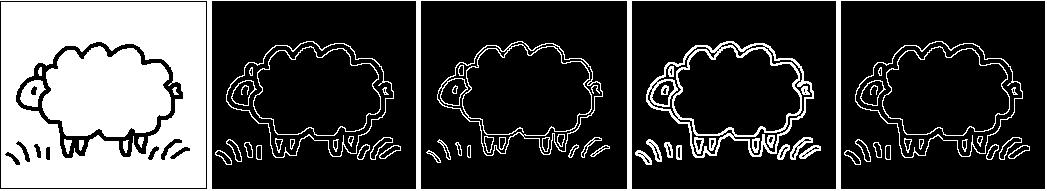# What is the Difference Between Mathematical Morphology Filters and Convolution Filters?What is the Difference Between Mathematical Morphology Filters and Convolution Filters?

Convolution filters generate output images in which the brightness value at a particular pixel depends on the weighted sum (i.e. linear combination) of the brightness of the neighboring pixels.

Mathematical morphology filters on the other hand perform nonlinear processing on images. These filters depend only on the relative ordering of pixel values as opposed to their numerical values. This property of mathematical morphology filters makes them really good when applied to binary images (a digital image that can only have two possible values for each pixel).

## Types of Convolution and Mathematical Morphology Filters

This page at Harrisgeospatial.com has a good overview of the different convolution filters and morphology filters.

The standard convolution filters are:

• High Pass
• Low Pass
• Laplacian
• Directional
• Gaussian Low Pass
• Gaussian High Pass
• Median
• Sobel
• Roberts
• User-Defined Convolution

The standard mathematical morphology filters are:

• Dilation
• Erosion
• Opening
• Closing